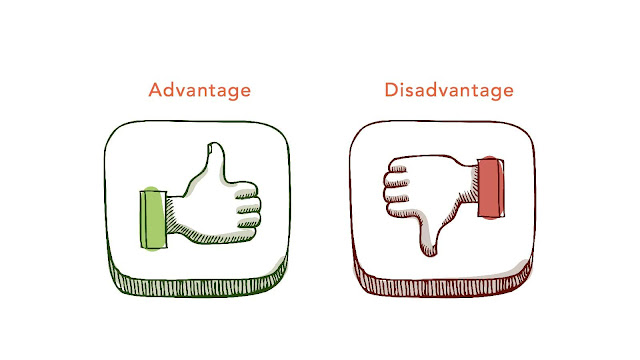# HSSlive: Plus One & Plus Two Notes & Solutions for Kerala State Board

## Monday, March 13, 2023Advantages And Disadvantages Of 2D Rectangular Matrix In Matlab

## What is 2D Rectangular Matrix In Matlab?

In MATLAB, a 2D rectangular matrix is a built-in data type that represents a two-dimensional array of numerical or logical values. It is called a rectangular matrix because it has a fixed number of rows and columns, and each row has the same number of columns.

You can create a 2D rectangular matrix in MATLAB using the `zeros`, `ones`, or `rand` functions, among others. For example, to create a 2D matrix with 3 rows and 4 columns, you can use the following code:

`A = zeros(3, 4); `

This will create a 3x4 matrix filled with zeros. You can also create a matrix with random values using the `rand` function, like this:

`B = rand(2, 5); `

This will create a 2x5 matrix with random values between 0 and 1.

Once you have created a matrix, you can perform various operations on it, such as indexing, slicing, and mathematical operations. For example, to access the value in the second row and third column of the matrix A, you can use the following code:

`A(2, 3) `

This will return the value in the second row and third column of A.

Overall, a 2D rectangular matrix in MATLAB is a powerful tool for working with numerical and logical data, and can be used for a wide range of applications such as data analysis, image processing, and simulation.

## Advantages of 2D Rectangular Matrix In Matlab

In MATLAB, a 2D rectangular matrix is a built-in data type known as a matrix. Here are some features and advantages of using a 2D rectangular matrix in MATLAB:

1. Easy to create and manipulate: In MATLAB, you can create a 2D rectangular matrix using the `zeros`, `ones`, or `rand` functions, among others. You can also manipulate the matrix using built-in functions and operators such as `+`, `-`, `*`, and `/`.

2. Powerful indexing and slicing: MATLAB provides powerful indexing and slicing capabilities for matrices, including the ability to use logical indexing and advanced indexing techniques.

3. Rich support for mathematical operations: MATLAB has a rich collection of built-in functions and toolboxes for mathematical operations, including matrix operations. This makes it easy to perform complex mathematical operations on matrices.

4. High performance: MATLAB is a compiled language and is highly optimized for matrix operations. This makes it a fast and efficient language for working with large matrices and performing complex calculations.

5. Interactive environment: MATLAB provides an interactive environment for working with matrices, including a command-line interface and a graphical user interface. This makes it easy to experiment with matrices and visualize results in real-time.

6. Versatile representation of data: A 2D rectangular matrix is a versatile representation of data in MATLAB, and can be used to represent a wide range of data structures such as tables, images, graphs, and more.

Overall, a 2D rectangular matrix in MATLAB is a powerful tool for working with data and performing complex mathematical operations. Its powerful indexing and slicing capabilities, rich support for mathematical operations, and high performance make it a popular choice for scientific and engineering applications.

## Disadvantages of 2D Rectangular Matrix In Matlab

Here are some potential disadvantages of using a 2D rectangular matrix in MATLAB:

1. High cost: MATLAB is a proprietary software, and its license can be expensive for individuals or small organizations. This can make it difficult to access for some users.

2. Steep learning curve: MATLAB has a steep learning curve for beginners, especially for those without prior programming experience. The language has its own syntax and programming concepts that can be difficult to grasp at first.

3. Limited memory: MATLAB's memory management can be limiting for very large matrices, especially when working on 32-bit systems. This can lead to memory errors and slow performance for large-scale applications.

4. Limited support for parallel computing: MATLAB's support for parallel computing is limited compared to other languages like Python or C++. This can make it challenging to perform computations on large matrices in parallel.

5. Limited integration with other tools: While MATLAB has a wide range of built-in functions and toolboxes, its integration with other tools and programming languages can be limited. This can make it challenging to integrate MATLAB with other data analysis tools.

6. Limited flexibility for non-matrix data: While 2D rectangular matrices are a powerful tool for many applications, MATLAB's focus on matrices can limit its flexibility for working with other types of data structures.

Overall, while 2D rectangular matrices are a powerful tool in MATLAB, the high cost of the software, steep learning curve, limited memory management, and limited support for parallel computing can be potential drawbacks for some users.

Share: